### Vyberte jazyk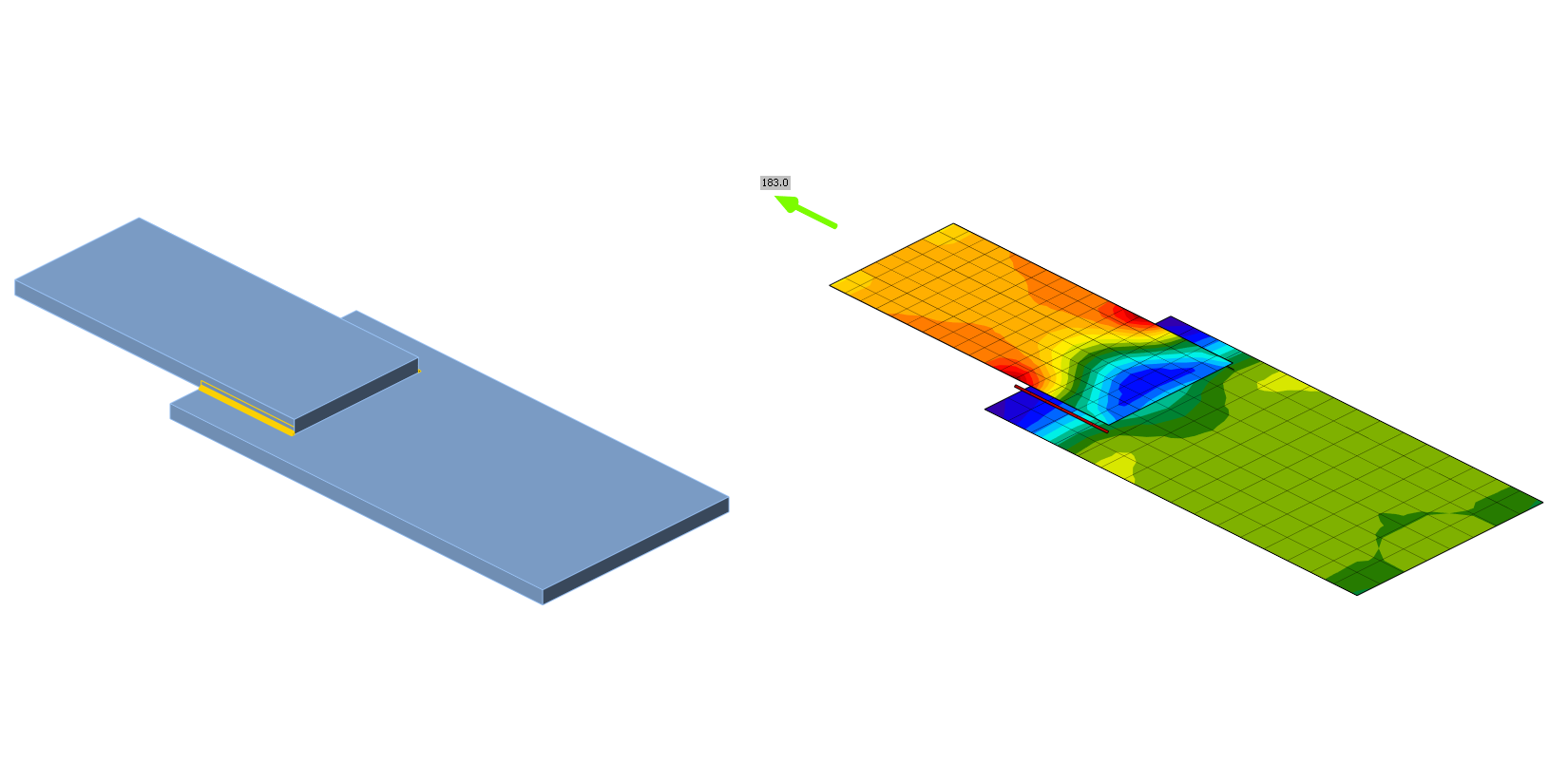# Fillet weld in lap joint

This article is a selected chapter from book Component-based finite element design of steel connections by prof. Wald et al. The chapter is focused on verification of welds.
$$DescriptionThe object of this chapter is verification of component-based finite element method (CBFEM) of a fillet weld in a lap joint with component method (CM). Two plates are connected in three configurations, namely with a transverse weld, with a longitudinal weld, and a combination of transverse and longitudinal welds. The length and throat thickness of the weld are the changing parameters in the study. The study covers long welds whose resistance is reduced due to stress concentration. The joint is loaded by a normal force.Analytical modelThe fillet weld is the only component examined in the study. The welds are designed to be the weakest component in the joint. The weld is designed according to EN 1993-1-8:2005. The design resistance of the fillet weld is determined using the Directional method given in section 4.5.3.2 in EN 1993-1-8:2005. The available calculation methods for checking the strength of fillet welds are based upon simplifying assumption that stresses are uniformly distributed within a throat section of a fillet weld. Uniform distribution of stress is assumed in a throat section of a weld, leading to the normal stresses and shear stresses shown in Fig. 4.1.1, as follows: σ⊥ is the normal stress perpendicular to the throat section; σ∥ is the normal stress parallel to the axis of the weld in its cross-section; τ⊥ is the shear stress (in the plane of the throat section) perpendicular to the axis of the weld; τ∥ is the shear stress (in the plane of the throat section) parallel to the axis of the weld. The normal stress σ∥ parallel to the axis is not considered when verifying the design resistance of a weld.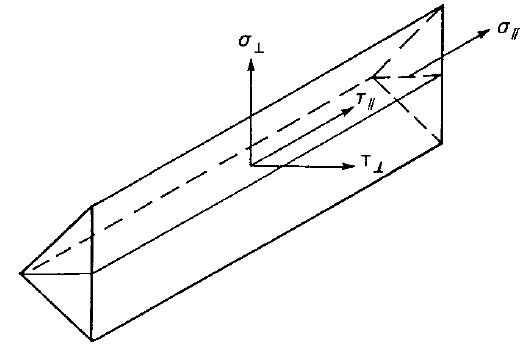Fig. 4.1.1 Stresses in a throat section of a fillet weldThe design resistance of the fillet weld will be sufficient if the following are both satisfied:$\sqrt{\sigma_{\perp}^2 + 3 ( \tau_{\perp}^2 + \tau_{\perp}^2 )} \le \frac{f_u}{\beta_w \gamma_{M2}}$$\sigma_{\perp} \le \frac{0.9 f_u}{\gamma_{M2}}$In lap joints longer than 150 a, the reduction factor βLw1 is given by:$$\beta_{Lw,1} = 1.2 - \frac{0.2 L_j}{150 a}$$ but $$\beta_{Lw,1} \le 1.0$$Overview of the considered examples and the material properties is given in Tab. 4.1.1. The weld configurations T is for transverse, P for parallel weld, and TP for a combination of both; see the geometry in Fig. 4.1.2. The sensitivity of two parameters, weld length and effective throat thickness, was investigated.Tab. 4.1.1 Overview of examples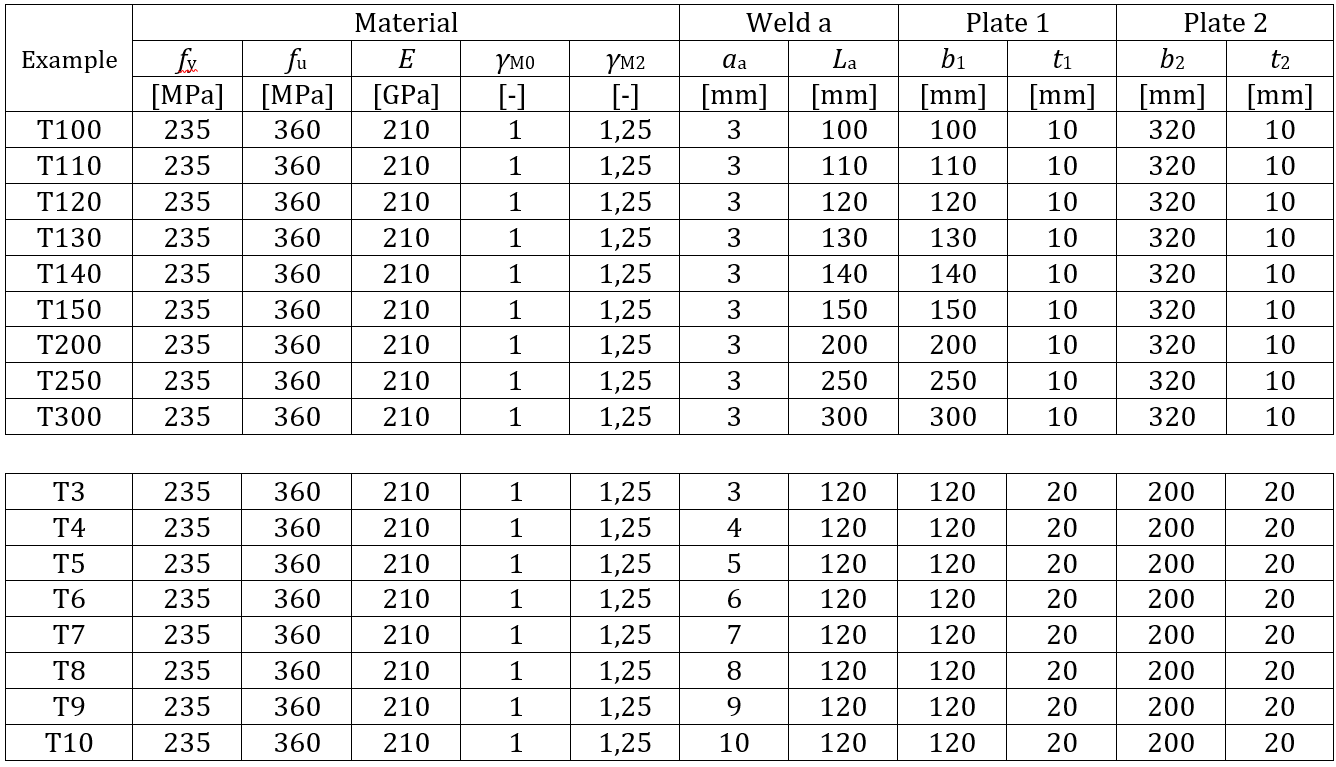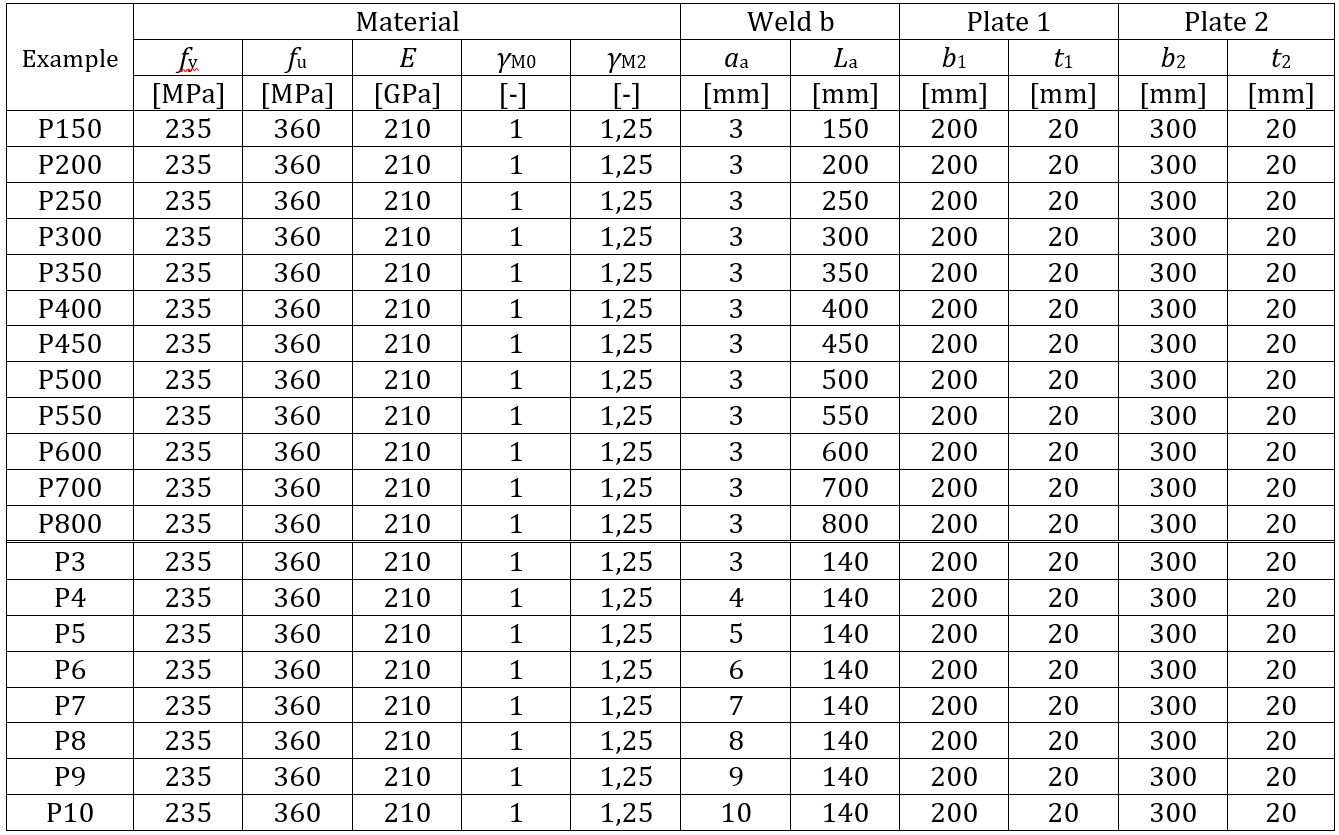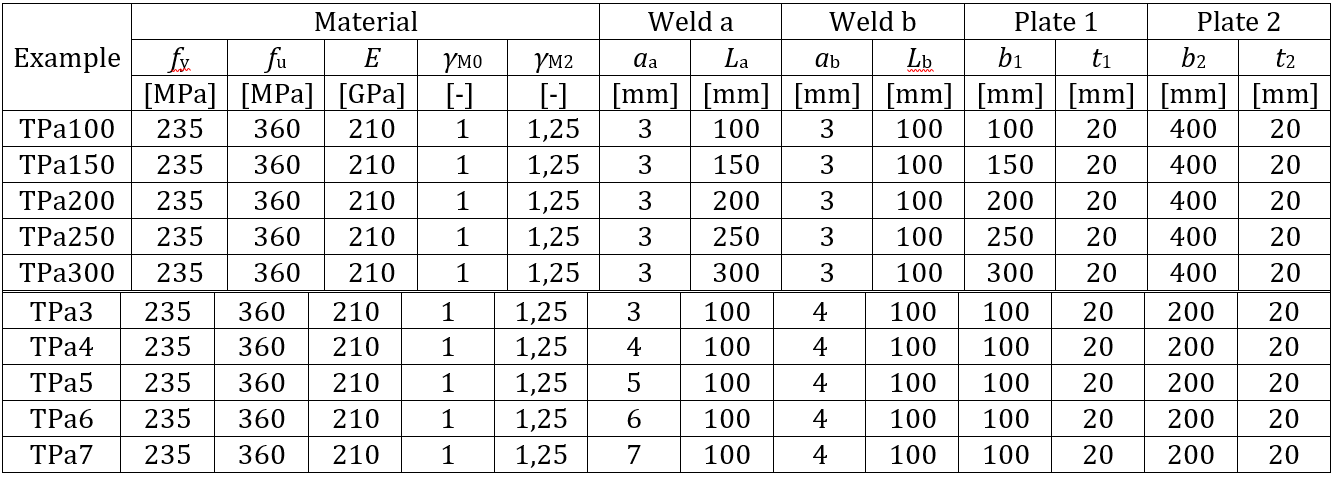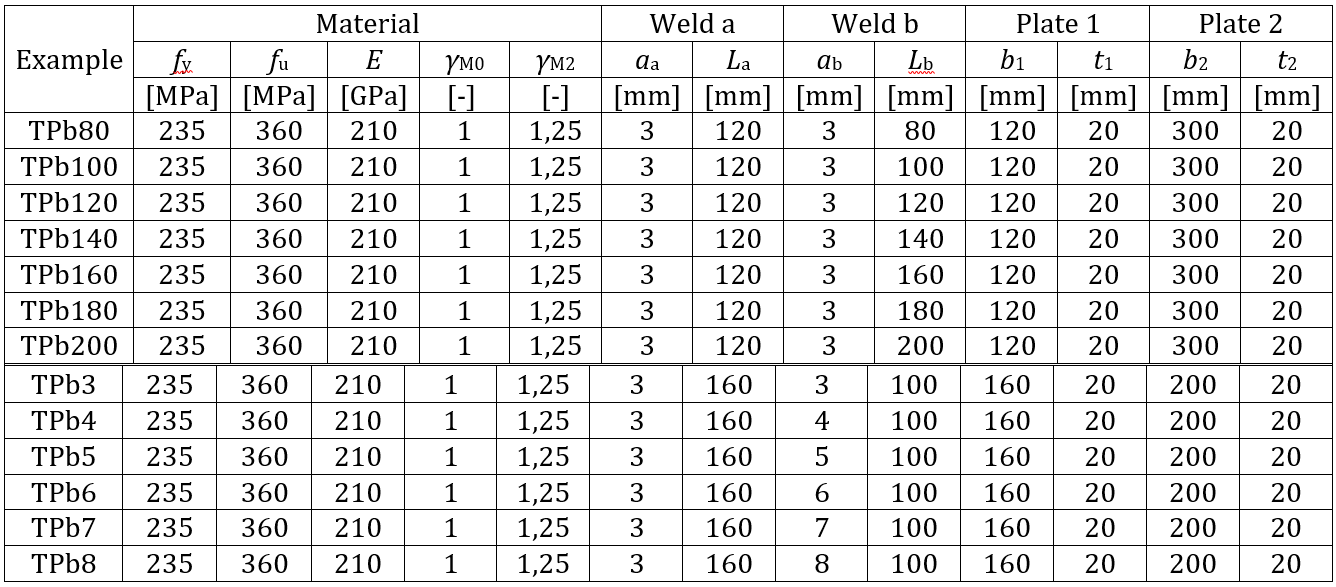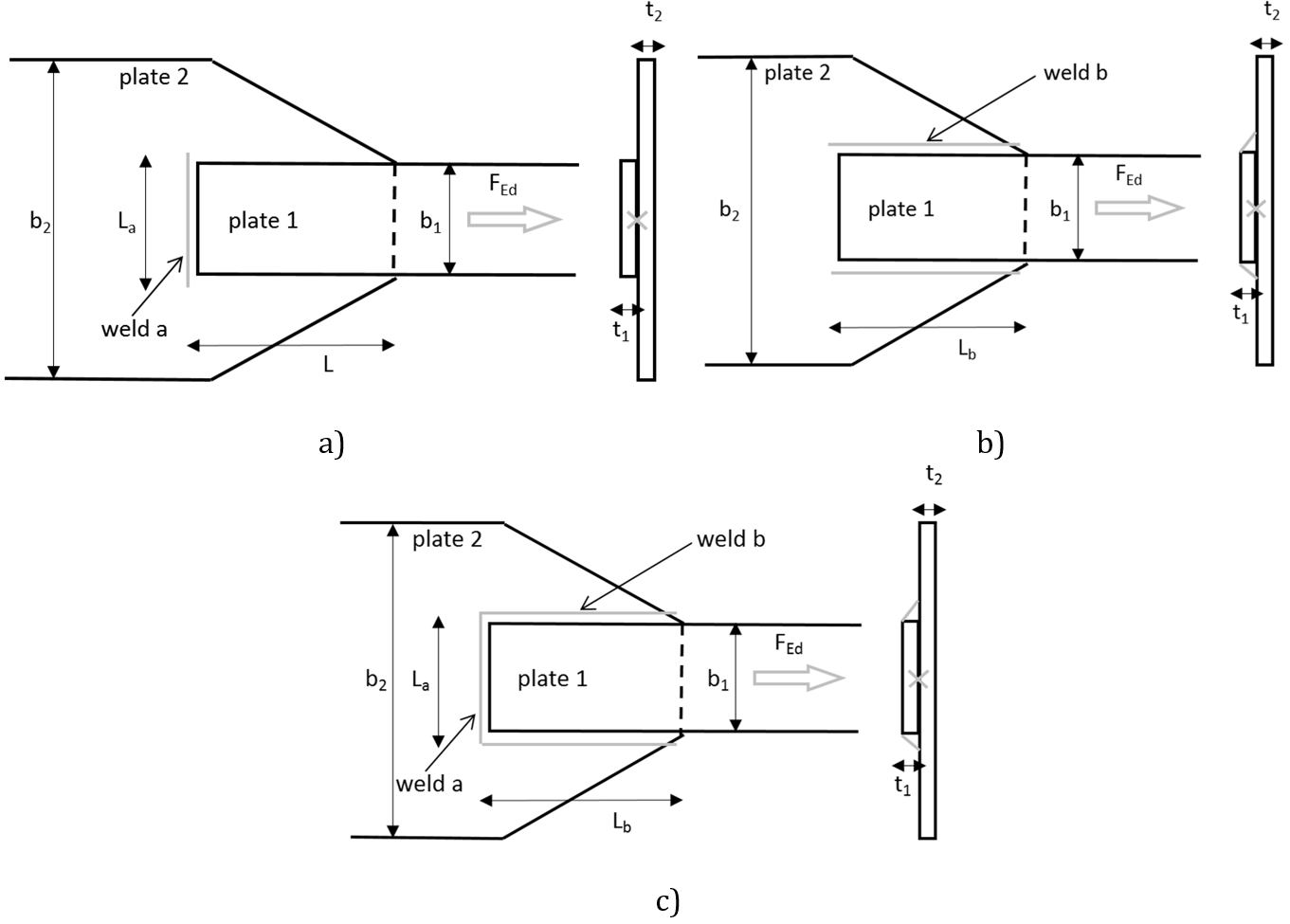Fig 4.1.2 Joint geometry with dimensions: a) Transverse weld b) Parallel weld c) Combination of transverse and parallel weldNumerical modelThe weld component in CBFEM is described in Chapter 3.4. Nonlinear elastic-plastic material is used for welds in this study. The limiting plastic strain is reached in the longer part of the weld, and stress peaks are redistributed.Verification of resistanceDesign weld resistance calculated by CBFEM is compared with the results of CM. The results are presented in Tab. 4.1.2. The study is performed for two parameters, length of the weld and effective throat thickness, in three weld configurations: for the transverse weld, parallel welds, and a combination of transverse and parallel welds.Tab. 4.1.2 Comparison of CBFEM and CM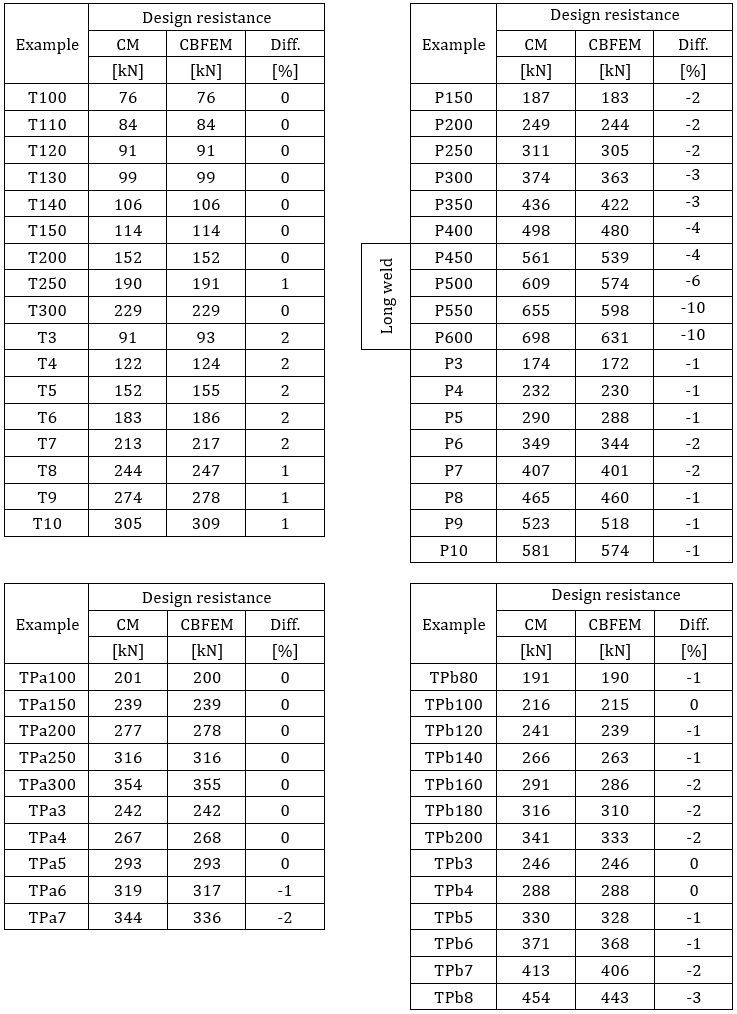Results of CBFEM and CM are compared, and a sensitivity study is presented. The influence of weld length and effective throat thickness on the design resistance of the transverse weld, parallel welds, and a combination of both welds is shown in Fig. 4.1.3, 4.1.4, and 4.1.5, respectively. The sensitivity study shows good agreement for all weld configurations.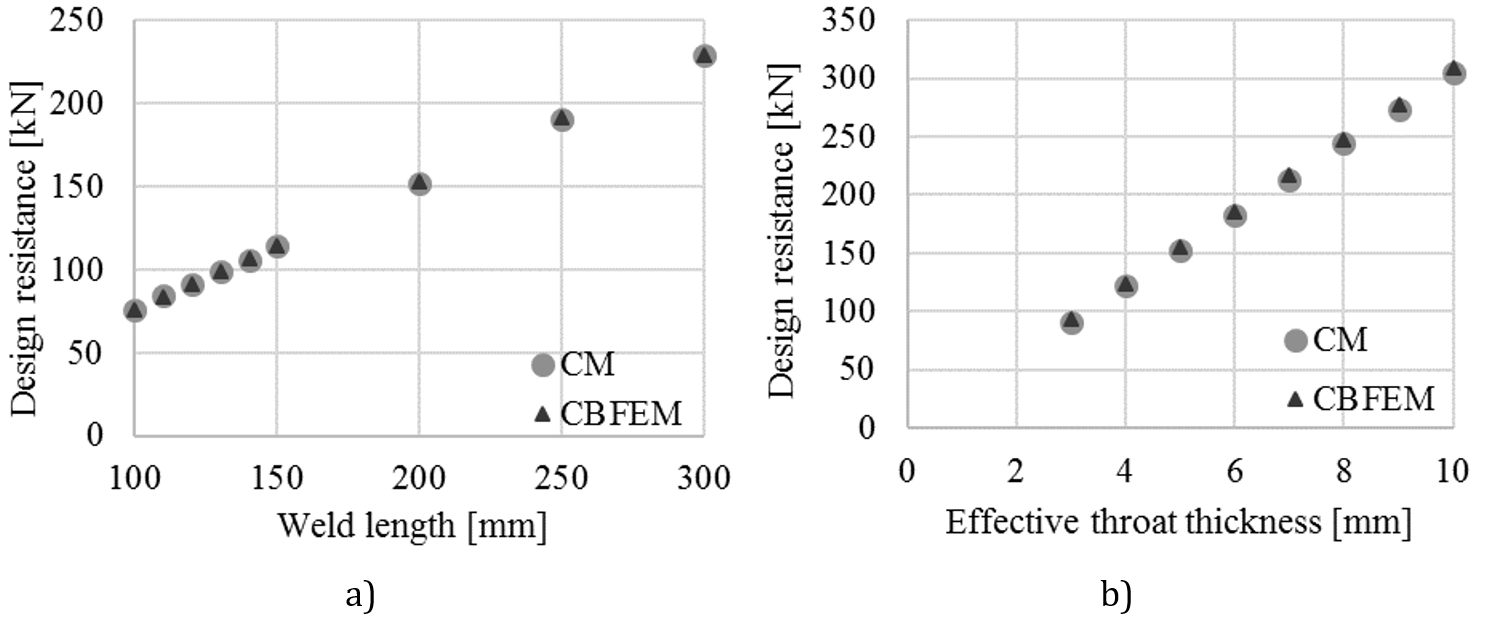Fig. 4.1.3 Parametric study of transverse weld a) Weld length b) Effective throat thickness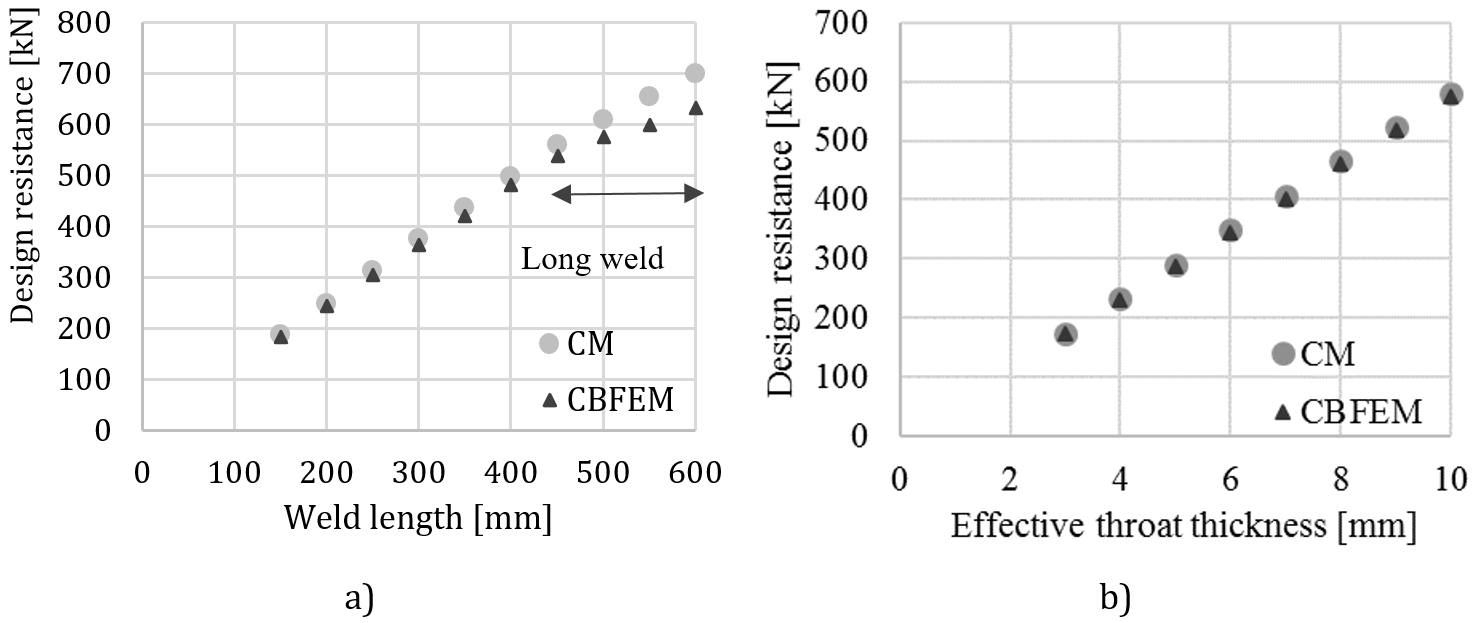Fig. 4.1.4 Parametric study of parallel weld a) Weld length b) Effective throat thickness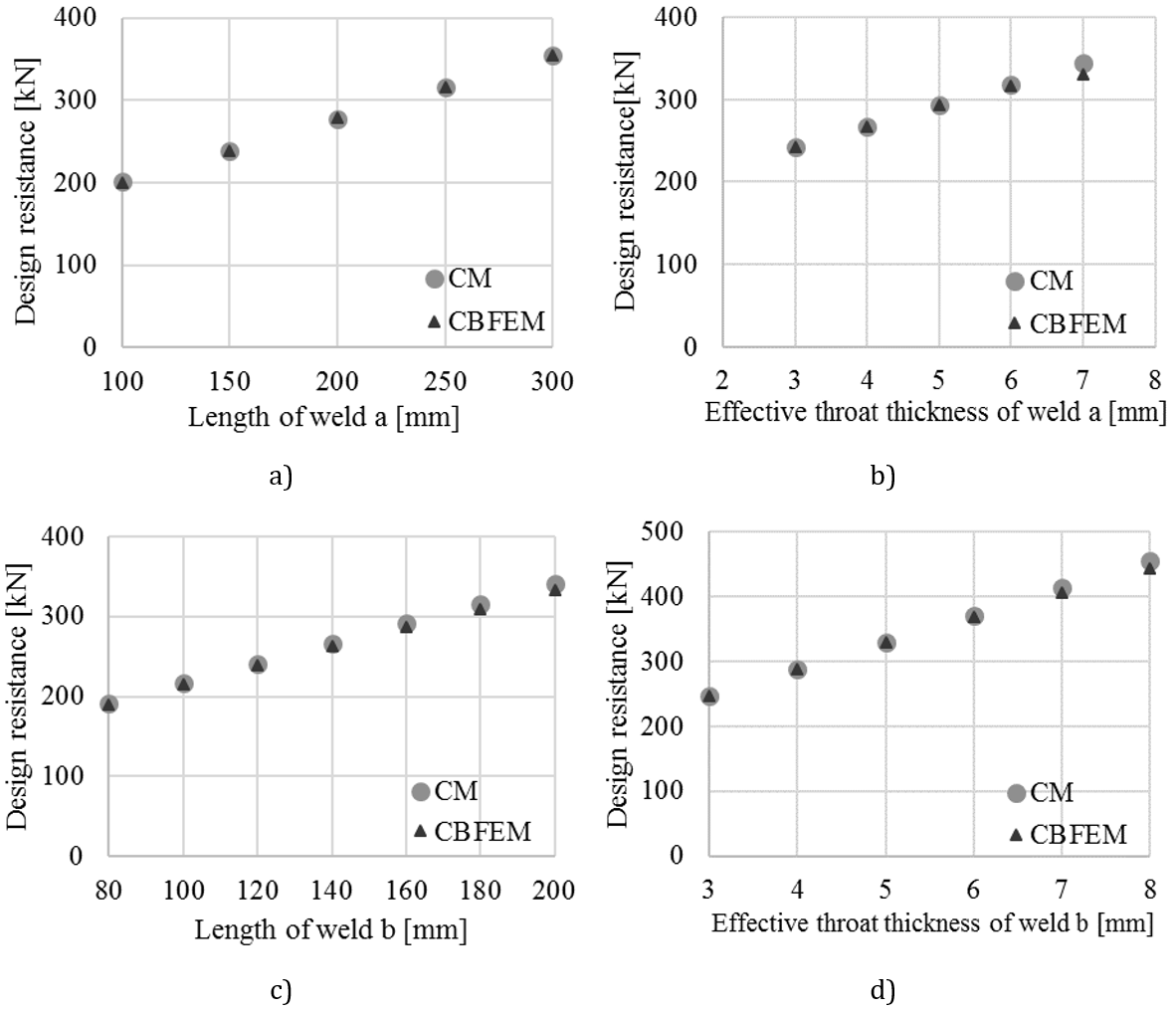Fig. 4.1.5 Parametric study of a combination of transverse and parallel weld: a) Length of weld a, b) Effective throat thickness of weld a, c) Length of weld b, d) Effective throat thickness of weld bTo illustrate the accuracy of the CBFEM model, the results of the sensitivity study are summarized in a diagram comparing CBFEM and CM design resistance; see Fig. 4.1.6. The results show that the difference between the two calculation methods is in all cases below 10 %.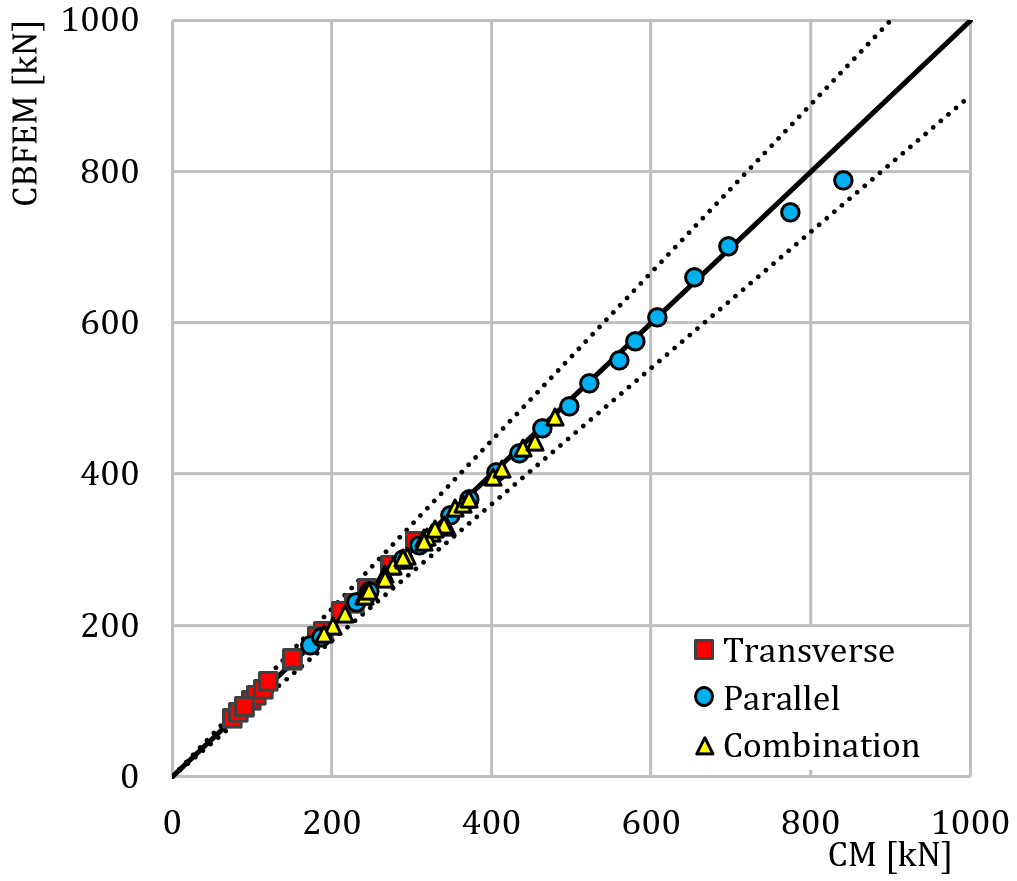Fig. 4.1.6 Verification of CBFEM to CMBenchmark exampleInputsPlate 1 Thickness t2 = 20 mm Width b2 = 300 mm Offset ez = −20 mm Steel S235 Plate 2 Thickness t1 = 20 mm Width b1 = 200 mm Steel S235 Model type N-Vy-Vz Weld, parallel fillet welds see Fig. 4.1.7. Throat thickness ab = 3 mm Weld length Lb = 150 mm Output Design resistance in tension FRd = 183 kN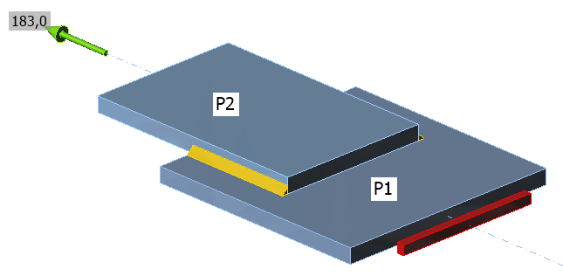Fig. 4.1.7 Benchmark example for the welded lap joint with parallel fillet welds Vzorové projekty Otevřít v aplikaci Viewer Stáhnout$$

## Vyzkoušejte program IDEA StatiCa

Stáhněte si zdarma zkušební verzi na 14 dní.